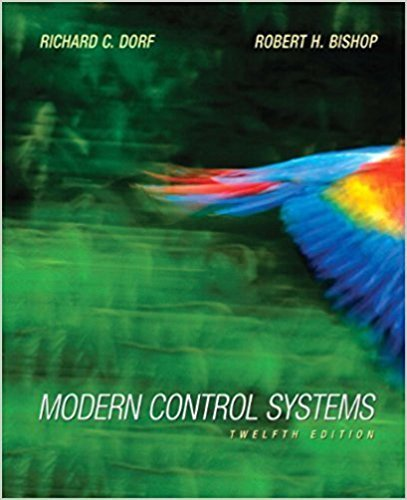Modern Control Systems - 12 Edition - Chapter 11 - Problem Dp11.3
Register Now

Join StudySoup

Get Full Access to Modern Control Systems - 12 Edition - Chapter 11 - Problem Dp11.39780136024583

# Consider the feedback system depicted in Figure DPI 1.3. The system model is given by

Modern Control Systems | 12th Edition

Problem DP11.3

Consider the feedback system depicted in Figure DPI 1.3. The system model is given by x(/) = Ax(f) + BK(/) y{t) = Cx(r) where A = 0 10.5 1 11.3 j ,B = 0 [0.55_ ,C = [1 0].

Accepted Solution
Step-by-Step Solution:
Step 1 of 3

th STAT 3600 Reference: Chapter 1 of Devore’s 8 Ed. Maghsoodloo Definition. A population is a collection (or an aggregate) of objects or elements that, generally, have at least one characteristic in common. If all the elements can be well defined and placed (or listed) onto a frame from which the sample can be drawn, then the population is said to be concrete and existing; otherwise, it is a hypothetical, conceptual, or a virtual population. Example 1. (a) All Auburn University students (N  29000 members on 2 campuses). Here the frame may be AU Telephone Directories. (b) All households in the city of Auburn. Again the frame can be the Auburn-Opelika Tel. Directory. (c) All AU COE (College of

###### Chapter 11, Problem DP11.3 is Solved

Step 2 of 3

Step 3 of 3

Unlock Textbook Solution